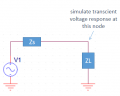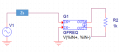# how to model a frequency dependent component in SPICE

#### shengwuei

Joined Aug 22, 2008
26
Hi Sir,

I've seen this, use "EFREQ" component to model a frequency dependent component in PSpice :
How to use EFREQ component in PSpice

In my case, I had used a vector network analyzer to measure the impedance(ZL) of a component on my circuit, for example :

 Frequency (Hz) real part (Ohm) imaginary part (Ohm) 1000 10 20 2000 30 -40 3000 50 -60 ... ... ...

And I would like to simulate the transient response waveform on ZL, as below (given Zs is known)To my knowledge, "EFREQ" is a voltage-to-voltage transfer component, and "GFREQ" is a voltage-to-current transfer component, and since impedance is equal to voltage divided by current, may I use a "GFREQ" component to model the measured impedance, as the following steps :
1. take the reciprocal of the measured impedance to transfer to conductance
2. set the frequency response of the conductance to a "GFREQ" component
3. place a resistor(R2) on the output of GFREQ.

Then if I observe the voltage difference across R2, does it represent the transient voltage response of ZL ?If I were wrong, is there any suggestion on this ?